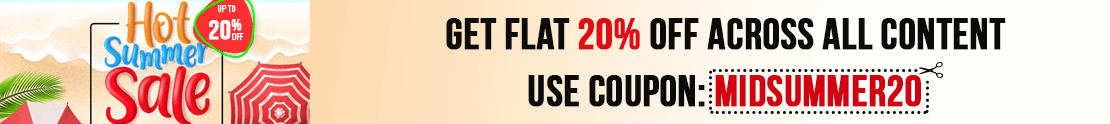# User ForumSubject :IMO    Class : Class 5

Class : Class 8

## Ans 2:

Class : Class 4
well the increase in height took place BETWEEN june and july so pls do explain which one shall i take

## Ans 3:

Class : Class 6
If it is taken in the first month then it should be July..........................I think...................

## Ans 4:

Class : Class 5
What happened to July?

Class : Class 7
C

## Ans 6:

Class : Class 6
oh my god. you have posted the easiest question in science olympiad foundation for grade 5 . the ans is july

## Ans 7:

Class : Class 7
yes, answer is C as the height was increased in June, not in July. The measure was taken on June 1st as mentioned. When you will read the question carefully, It will become really simple.Its not arduous at all.

Class : Class 7

Class : Class 7

## Ans 10:

Class : Class 6
if you say that even may can be the answer

## Ans 11:

Class : Class 8
C is the answer I am sure!

## Ans 12:

Class : Class 5
D is the answer as increase in height in April is 10 cm.,May is 12 cm., June is 11 cm., and July is 13 cm. So, the most increase in height took place in July.

## Ans 13:

Class : Class 6
oh my god. you have posted the easiest question in science olympiad foundation for grade 5 . the ans is july

Class : Class 5

## Ans 15:

Class : Class 6
It is actually c because u should not count the cm in the month but in the before months increasing

Class : Class 6

## Ans 17:

Class : Class 7
If anyone says July is the answer than you have told the wrong answer ,the answer is June

## Ans 18:

Class : Class 5
D is the answer as increase in height in April is 10 cm.,May is 12 cm., June is 11 cm., and July is 13 cm. So, the most increase in height took place in July.

## Ans 19:

Class : Class 6
It is d because it is wrong in solution

Subject :IMO    Class : Class 5

Class : Class 5
c reflex angle

## Ans 2:

Class : Class 5
I want to go with answer c) Reflex Angle

Class : Class 4

Class : Class 8
For me it is (C)

Class : Class 6
Reflex on board

## Ans 6:

Class : Class 6
How Reflex angle??? it is a right angle.

## Ans 7:

Class : Class 5
I know you have this question from book of mtg imo class 5 chapter angles and question no. 10

Class : Class 5

Class : Class 5
it is c)

Class : Class 6
it should be b

## Ans 11:

Class : Class 7
It should be Option C) because Obtuse Angle is always less than 180 but more than 90 and X shown in the figure is greater than 180 degree so it should be Reflex Angle

Subject :IMO    Class : Class 5

## Ans 1:

Class : Class 6
(a) 3 hours 45 min 15 seconds

Subject :IMO    Class : Class 8

Class : Class 9
C

Class : Class 8
And is option C

## Ans 3:

Class : Class 9
LET THE NUMBER BE X AND Y X-Y=1 X=Y+1 GIVEN X+Y=25 EQUATE X AS Y+1 Y+1+Y=25 2Y+1=25 2Y=24 Y=12 X=25-Y=13 ANSWER IS C-12,13

## Ans 4:

Class : Class 8
And is option C

Subject :IMO    Class : Class 8

Subject :IMO    Class : Class 5

Subject :IMO    Class : Class 5

Class : Class 8
B

## Ans 2:

Class : Class 6
Be sure that you are clear and tell me the version of your computer as a question in general subject right now. also you can send it as an answer in this post

Subject :IMO    Class : Class 7

Class : Class 9

Class : Class 7

Class : Class 9

## Ans 4:

Class : Class 9
TOTAL AREA OF FIGURE= 1/2 PI R^2= 1/2*14*14*22/7=308CM^2 AREA OF WHITE ARE= PI R^2 22/7*7*7=154 cm^2 THEREFORE RE O SHADED REGION= 308-154=154 CM^2 OPTION C IS CORRECT

## Ans 5:

Class : Class 7

Subject :IMO    Class : Class 5

Class : Class 8
D

## Ans 2:

Class : Class 4
Letâs see what pattern is being followed by the numbers in the above grid. Letâs take the first column â 7, 81, 2 If we add 1st and 3rd number = 7 2 = 9 And if we multiply 9 by 9 itself = 9 x 9 = 81. 81 is the 2nd number in the first column. Letâs see if the numbers in second column follow the same pattern. 9, 169, 4 We add 1st and 3rd number = 9 4 = 13 We multiply 13 by 13 itself = 13 x 13 = 169. 169 is the 2nd number in the second column. That means the numbers of third column will follow the same pattern. 11, ?, 6 We add 1st and 3rd number = 11 6 = 17 We multiply 17 by 17 itself = 17 x 17 = 289. 289 will be the missing number in the third column. So, the correct answer is Option D.

## Ans 3:

Class : Class 6
It is D because 11 6=17and 17x17 is 289.